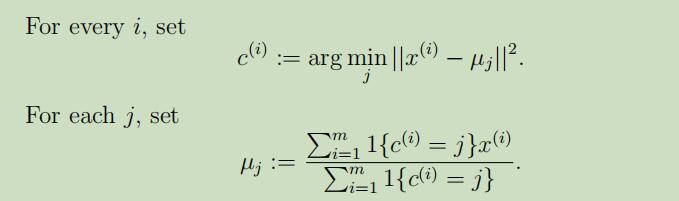# 吴恩达Stanford机器学习公开课（十二）笔记

## Lecture 12 - k-means，Mixtures of Gaussians，EM algorithm

Posted by Yunlongs on May 22, 2019

# Lecture 12 - k-means，Mixtures of Gaussians，EM algorithm

## K-means

1. 随机初始化聚类中心$\mu_{1}, \mu_{2}, \dots, \mu_{k} \in \mathbb{R}^{n}$
2. 重复直到收敛：K-means算法一定能保证收敛吗？ 是的。现在让我们定义失真函数（distortion function）如下：## 混合高斯模型（mixture of Gaussians）

事实上，当我们知道了$z^{(i)}$后，参数的估计就和以前学过的高斯判别分析模型中的参数估计大致一样

## EM算法原理详细解释

### Jensen’s 不等式

Remark： 当且仅当$-f$为[严格]凹函数时，$f$是[严格]凸函数（$f^{\prime \prime}(x) \leq 0$ or $H \leq 0$）。当$f$为凸函数时，Jensen’s 不等式仍然成立，不过结果变成了$\mathrm{E}[f(X)] \leq f(\mathrm{E} X)$

### EM算法原理

Remark：如果我们定义### EM算法参数更新过程推导

$\Sigma_{j}$的推导过程见作业。